## MP Board Class 8th Maths Solutions Chapter 2 Linear Equations in One Variable Ex 2.5

Solve the following linear equations.

Question 1.
$$\frac{x}{2}-\frac{1}{5}=\frac{x}{3}+\frac{1}{4}$$
Solution: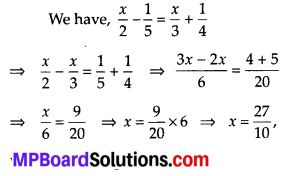which is the required solution.Question 2.
$$\frac{n}{2}-\frac{3 n}{4}+\frac{5 n}{6}=21$$
Solution: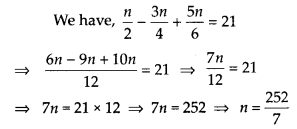⇒ n = 6, which is the required solution.

Question 3.
$$x+7-\frac{8 x}{3}=\frac{17}{6}-\frac{5 x}{2}$$
Solution: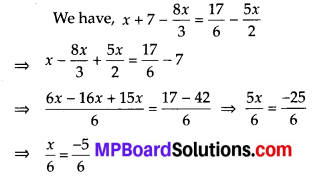⇒ x = -5, which is the required solution.Question 4.
$$\frac{x-5}{3}=\frac{x-3}{5}$$
Solution: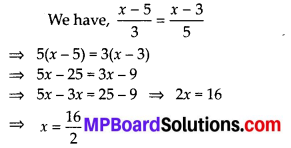⇒ x = 8, which is the required solution.

Question 5.
$$\frac{3 t-2}{4}-\frac{2 t+3}{3}=\frac{2}{3}-t$$
Solution: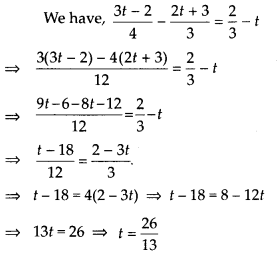⇒ t = 2, which is the required solution.Question 6.
$$m-\frac{m-1}{2}=1-\frac{m-2}{3}$$
Solution: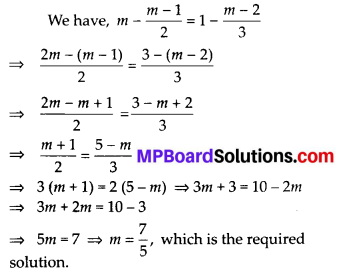Simplify and solve the following linear equations.

Question 7.
3(t – 3) = 5(2t + 1)
Solution:
We have, 3(t – 3) = 5(2t + 1)
⇒ 31 – 9 = 101 + 5
⇒ – 9 – 5 = 101 – 31 ⇒ -14 = 71
⇒ 1 = – 2, which is the required solution.Question 8.
15(y – 4) – 2(y – 9) + 5(y + 6) = 0
Solution:
We have,
15(y – 4) – 2(y – 9) + 5(y + 6) = 0
⇒ 15 y – 60 – 2y + 18 + 5y + 30 = 0
⇒ 18y – 12 = 0 ⇒ 18y = 12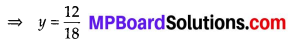⇒ y = $$\frac{2}{3}$$, which is the required solution.

Question 9.
3(5z – 7) – 2(9z -11) = 4(8z – 13) – 17
Solution:
We have,
3(5z – 7) – 2(9z -11) = 4(8z – 13) – 17
⇒ 15z – 21 – 18z + 22 = 32z – 52 – 17
⇒ 15z – 18z – 32z = 21 – 22 – 52 – 17 – 70
⇒ -35z = -70 ⇒ z = $$\frac{-70}{-35}$$
⇒ z = 2, which is the required solution.Question 10.
0.25 (4f- 3) = 0.05(10f – 9)
Solution:
We have, 0.25(4f – 3) = 0.05(10f – 9)
⇒ f – 0.75 = 0.5f – 0.45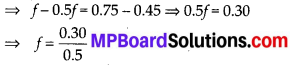⇒ f = 0.6, which is the required solution.